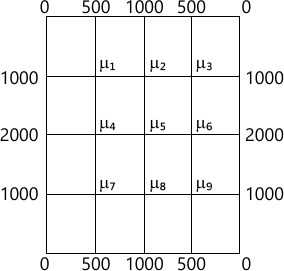MORE IN Engineering Mathematics 3
Total marks: --
Total time: --
INSTRUCTIONS
(1) Assume appropriate data and state your reasons
(2) Marks are given to the right of every question
(3) Draw neat diagrams wherever necessary

1 (a) Find Fourier series of f(x)=2Πx-x2 in [0, 2Π]. Hence deduce $\sum^{\infty}_1 \dfrac {1}{(2n-1)^2} = \dfrac {\pi^2}{8}$ sketch the graph of f(x).
7 M
1 (b) Find Fourier cosine series of $f(x)= \sin \left ( \dfrac {m\pi}{l} \right )x,$ where m is positive integer.
6 M
1 (c) Following table gives current (A) over period (T):
 A (amp) 1.98 1.30 1.05 1.30 -0.88 -0.25 1.98 t (sec) 0 T/6 T/3 T/2 2T/3 5T/6 T

Find amplitude of first harmonic:
7 M

2 (a) Find Fourier transformation of ea2x2 (-∞ -x2/3 is self reciprocal.
7 M
2 (b) Find Fourier cosine and sine transformation of $f(x)= \left\{\begin{matrix} x &0 6 M 2 (c) Solve integral equation \[ \int^\infty_{0} f(x) \cos sx dx = \left\{\begin{matrix} 1-s &0 7 M 3 (a) Find various possible of one dimensional wave equation \[ \dfrac {\partial^2 u}{\partial t^2} = c^2 \dfrac {\partial ^ 2 u}{\partial x^2}$ by separable variable method.
7 M
3 (b) Obtain solution of heat equation $\dfrac {\partial u}{\partial t^2} = c^2 \dfrac {\partial^2 u}{\partial t^2}$ subject to condition u(0,t)=0, u(l,t)=0, u(x,0)=f(x).
6 M
3 (c) Solve Laplace equation $\dfrac {\partial ^2 u}{\partial x^2}+ \dfrac {\partial^2 u}{\partial y^2} =0$ subject to condition u(0, y)=u (l, y)=u (x,0)=0; $u (x,a)=\sin \left ( \dfrac {\pi x}{l} \right )$
7 M

4 (a) The revolution (r) and time (t) are related by quadratic polynomial r=at2+bt+c. Estimate number revolution for time 3.5 units, given
 Revolution 5 10 15 20 25 30 35 Time 1.2 1.6 1.9 2.1 2.4 2.6 3
7 M
4 (b) Solve by graphical method, Minimize Z=20x1+10x2 under the constraints $2x_1+x_2 \ge 0; \ x_1+2x_2 \le 40; \ 3x_1+x_2 \ge 0; \ 4x_1+ 3x_2 \ge 60; \ x_1,x_2 \ge 0$
6 M
4 (c) A company produces 3 items A, B, C. Each unit of A requires 8 minutes, 4 minutes and 2 minutes of producing time on machine M1, M2 and M3 respectively. Similarly B requires 2, 3, 0 and C. Requires 3, 0, 1 minutes of machine M1, M2 and M3. Profit per unit of A, B and C are Rs.20, Rs.6 and Rs.8 respectively. For maximum profit, how many number of products A. B and C are to be produced? Find maximum profit. Given machine M1, M2 and M3 are available for 250, 100 and 60 minutes per day.
7 M

5 (a) By relaxation method, solve -x+6y+27z=85, 54x+y+z=110, 2x+15y+6z=72.
7 M
5 (b) Using Newton-Raphson method derive the iteration formula to find the value of reciprocal of positive number. Hence use to find 1/e upto 4 decimals.
6 M
5 (c) Using Power Rayleigh method find numerical largest Eigen value and corresponding Eigen vector for $\begin{bmatrix} 10 &2 &1 \\2 &10 &1 \\2 &-1 &10 \end{bmatrix}$ using (1, 1, 0)T as initial vector. Carry out 10 iterations.
7 M

6 (a) Fit interpolating polynomial for f(x) using divided difference formula and hence evaluate f(z), given f(0)= -5, f(1)=-14= -125, f(8)=-21, f(10)=355.
7 M
6 (b) Estimate t when f(t)=85 using inverse interpolation formula given:
6 M
6 (c) A solid of revolution is formed by rotating about x-axis the area between x-axis lines x=0, x=1 and curve through the points with the following co-ordinates.

by Simpson's 3/8th rule, find volume of solid formed.
7 M

7 (a) Using the Schmidt two-level point formula solve $\dfrac {\partial^2 u}{\partial x^2} = \dfrac {\partial u}{\partial t}$ under the conditions u(0,t)=u(1,t)=0; t?0; u(1,0)=sin Πx 0
7 M
7 (b) Solve the wave equation $\dfrac {\partial^2 u}{\partial t^2} = 4 \dfrac{\partial ^2 u}{\partial x^2}$ subject to u(0,t)=0. U(4, t)=0, u1(x,0)=0 and u(x,0)=x(4-x) by taking h=1, K=0.5
6 M
7 (c) Solve $\dfrac {\partial^2 u}{\partial x^2} + \dfrac {\partial^2 u}{\partial y^2} =0$ in the square mesh. Carry out 2 iterations.7 M

8 (a) State and prove recurrence relation of f-transformation hence find ZT(n), Z1(n2).
7 M
8 (b) Find ZT[30n0 cosh n()-sin (nA+0)+n].
6 M
8 (c) Solve difference equation un+2+6un+1+9un=n2n given u0=u1=0.
7 M

More question papers from Engineering Mathematics 3